# Textbooks

This page contains an information about some textbooks developed by our research workers. You can view the full list of textbooks (including textbooks written in Russian and Ukrainian) on this page.

▲  Differential and Integral Calculus for One Variable Functions. / L.V. Kurpa, T.V.Shmatko. Differential and Integral Calculus for One Variable Functions: Textbook. – Kharkiv: NTU KhPI:  2017. – 324 pages.Authors: Kurpa L.V,  Shmatko T.V.

This handbook includes theoretical material with all the necessary proof of the theorems for section of Higher Math “Differential and integral calculus for functions with one variable” Proposed materials correspond to education program from higher mathematics for technical universities related with point section. The handbook does not purport to address the presentation of complete course of higher mathematics, but to some extent compensates for the lack of English literature in the course of higher mathematics in the Ukraine.

The authors hope that the manual will help students in learning this section of Mathematics and will be useful for teachers who give lectures in English.

▲ Higher Mathematics. Problem solving and variants of typical calculations (Edited by Prof. Kurpa L.V.)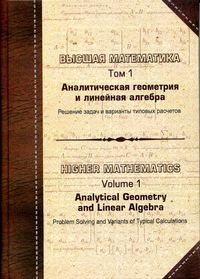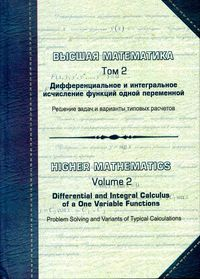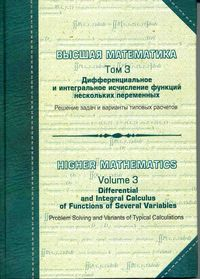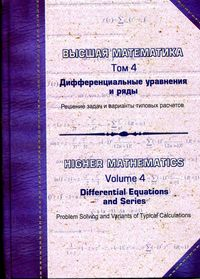The textbook “Higher Mathematics” in four volumes is based on materials which were published earlier in books “Вища математика” and “Higher mathematics” under editorship of prof. Kurpa L.V. presented in 2 volumes in 2002 and 2004. However, this edition is fundamentally overworked, extended, supplemented and given in 2 languages (Russian and English). For each theme only main theoretical knowledge is expounded in an organic form. The special consideration is given to methods of problem solving. It helps the student to understand theoretical materials more profoundly, teaches him to apply mathematical tools to solve concrete tasks. Individual tasks (30 variants) and also test questions and control tasks are presented to each theme. By means of these tasks student can check his preparedness to self-dependent carrying-out of individual tasks. Both test tasks and individual tasks are supplied with answers that will help the student to check the accuracy of the obtained solution. The given textbook is characterized by examples of tasks solved in Maple software, which text of programs are also presented. Taking into account modern requirements to engineer professional skills and the high level of information technology development, the authors have decided to include in the textbook primary information about one of the modern mathematical system “Maple”. The authors think that the parallel solution of many problems of higher mathematics by means of the package Maple and without them allows to check the solution obtained by student independently. Using these packages will set free an engineer of routine mathematical calculations and transformations in future. It will promote intensification and development of the creative approach to execute either problems. Therefore, the textbook contains tasks solved by means of the system “Maple” together with worked out programs. This textbook will be very useful not only for students from UIC but also for foreign students learning in Ukraine, Russia, Byelorussia. The authors are very grateful to many teachers that gave answers to individual tasks including E.S. Arkhipova, L.V. Bolotina, T.L. Kornil, L.P. Lemesheva, N.N. Yasnitskaya and others.

▲ Higher Mathematics Problem solving and variants of typical calculations. (Edited by Prof. Kurpa L.V.)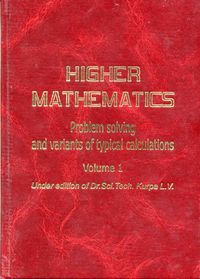The educational textbook includes theoretical material of main sections of higher mathematics: linear algebra, analytical geometry and calculus, fundamentals of differential calculus of function of several variables, multiple, curvilinear and surface integrals, field theory and sets, examples of solving typical problems and variants of typical calculations.

▲ Differential calculus for one variable functions.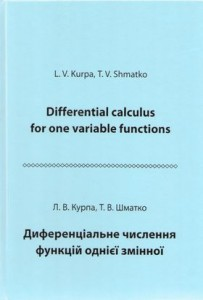Authors: L.V. Kurpa, T.V. Shmatko

▲ Differential and integral calculus for functions with several variablesAuthors: L.V. Kurpa, T.V. Shmatko

Theoretical material of differential calculus of several variables, multiple and curvilinear integrals is proposed The educational textbook includes problems that are recommended to solve as classwork and as homework. Variants of individual tests are created for each subject. For students and teachers of technical specialties.

▲ Elements of Linear Algebra and Analytic GeometryAuthor: G.V. Rudnyeva

The textbook “Elements of Linear Algebra and Analytic Geometry” presents a course of mathematics suitable for under-graduate students of the technical universities and has grown out of a course of lectures given by the author at National Technical University “Kharkov Polytechnic Institute” during the past four years to the students studying the mathematical courses in English.

▲ An introduction to probability theory and mathematical statistics.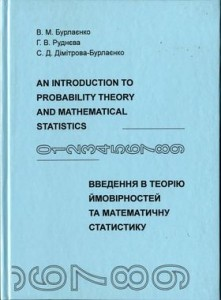Author: V.N.Burlayenko

This educational textbook contains theoretical material on the probability theory alongside mathematical statistics. Main theorems and statements are proven and main formulas are derived necessary to solve the practical problems. Variants of typical tasks on considered subjects are proposed tor individual work. Application of the material is illustrated by numerous examples and figures.

The textbook is recommended to students of technical specialties studying the course “Probability Theory and Mathematical Statistics” in English as well as foreign students and teachers of higher mathematics.

▲ Differential Equations and Series / L.V. Kurpa, O.S. Mazur, T.V. Shmatko: Textbook. – Kharkiv: NTU KhPI, 2013. – 288 pages.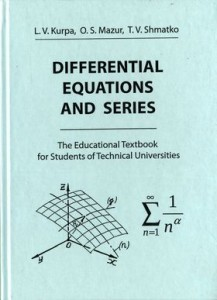Authors: L.V. Kurpa, O.S. Mazur, T.V. Shmatko

Theoretical material for study of differential equations and series is presented. The manual contains different tasks recommended for work as in the class so at work. Variants of practical tests are proposed for each topic. This book is for students of technical universities that use English for teaching higher mathematics.

▲ Normal Modes and Localization in Nonlinear Systems / Vakakis, A. F., Manevitch, L. I., Mikhlin, Y. v., Pilipchuk, V. N., and Zevin A. A.  Wiley, New York, 1996.Authors: A.F. Vakakis, L.I. Manevitch, Yu.V. Mikhlin, V.N. Pilipchuk,     A.A. Zevin

This landmark volume offers a completely new angle on the study of vibrations in discrete or continuous nonlinear oscillators. It describes the use of NNMs to analyze the vibrations of nonlinear systems and design systems with motion confinement properties.

Normal Modes and Localization in Nonlinear Systems features

• New and established mathematical techniques that can be used for more refined vibration and shock isolation designs of practical flexible structures
• Complete coverage of free and forced motions in systems with weak or strong nonlinearities, including results that cannot be captured with existing linear or quasi-linear techniques
• A new method for analyzing strongly nonlinear systems that permits perturbation analysis of systems with essential nonlinearities
• A theoretical link between nonlinear normal modes and standing solitary waves
• The first experimental verifications of nonlinear mode localization and nonlinear motion confinement in flexible engineering structures

You can read this book on the site …